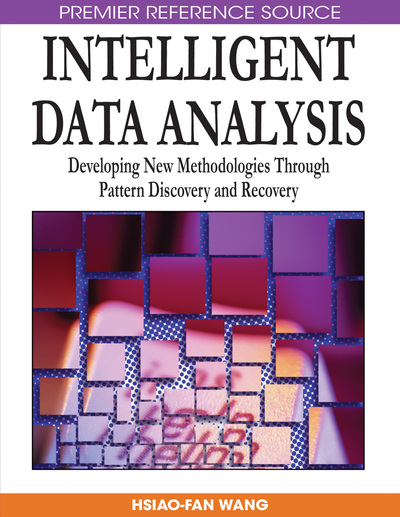# Exact Markov Chain Monte Carlo Algorithms and Their Applications in Probabilistic Data Analysis and Inference

Dominic Savio Lee (University of Canterbury, New Zealand)
Copyright: © 2009 |Pages: 23
DOI: 10.4018/978-1-59904-982-3.ch010
OnDemand PDF Download:
Available
\$37.50
No Current Special Offers

## Abstract

This chapter describes algorithms that use Markov chains for generating exact sample values from complex distributions, and discusses their use in probabilistic data analysis and inference. Its purpose is to disseminate these ideas more widely so that their use will become more widespread, thereby improving Monte Carlo simulation results and stimulating greater research interest in the algorithms themselves. The chapter begins by introducing Markov chain Monte Carlo (MCMC), which stems from the idea that sample values from a desired distribution f can be obtained from the stationary states of an ergodic Markov chain whose stationary distribution is f. To get sample values that have distribution f exactly, it is necessary to detect when the Markov chain has reached its stationary distribution. Under certain conditions, this can be achieved by means of coupled Markov chains—these conditions and the resulting exact MCMC or perfect sampling algorithms and their applications are described.
Chapter Preview
Top

## Markov Chain Monte Carlo

MCMC is based on the observation that the state of an ergodic Markov chain (see Appendix to this chapter for a glossary of Markov chain properties) will eventually converge to a stationary distribution, no matter which state the chain starts in. Thus, to obtain a sample from a desired distribution f, an ergodic Markov chain with f as its stationary distribution can be constructed and then run till it is stationary. The required sample values are given by the states of the stationary chain. Letrepresent a sequence of states for an ergodic Markov chain and suppose that it reaches its stationary distribution f after transition T, then a dependent sample with distribution f is given by. This sample can be used in the same way as an independent sample for the estimation of expectations; by the strong law of large numbers, the sample average for a measurable function h will converge, almost surely, to the expectation under f:

Note, however, that the sample variance cannot simply be used as an estimate of Monte Carlo standard error because of the dependency within the sample (Kass, Carlin, Gelman, & Neal, 1998). Instead, the autocorrelation in the sample must be estimated and used to estimate the standard error. If an independent sample is required, multiple chains with independent starting states must be used.

Generic algorithms are available that allow an ergodic Markov chain with a specified stationary distribution to be constructed easily. Many of these algorithms can be regarded as variants of the Metropolis-Hastings algorithm, commonly attributed to Metropolis, Rosenbluth, Rosenbluth, Teller, and Teller (1953) and Hastings (1970). Letrepresent the uniform distribution on the interval (0, 1).

## Complete Chapter List

Search this Book:
Reset